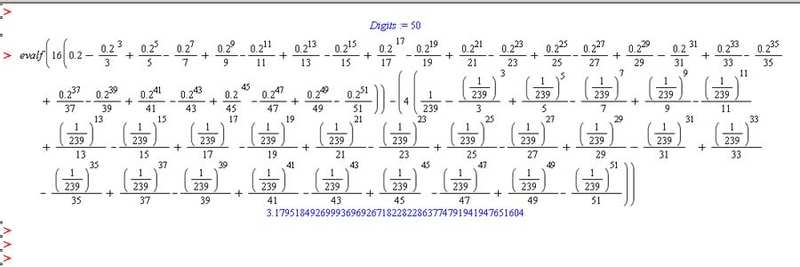# Help Calculating Pi using Arctangent formula

• PolyFX

## Homework Statement

Using the Arctangent formula
pi = 16 * arctan (1 / 5) - 4*arctan(1 / 239) to calculate the value of pi to 53 significant digits.

## Homework Equations

The power series of arctangent(x) is = x − x^3/3 + x^5/5 − x^7/7 + x^9/9...

## The Attempt at a Solution

http://tinypic.com/r/fy1tax/5I attempted this question on MAPLE 13(shown above), but I get an obvious incorrect answer 3.17... instead of 3.14...

Am i using the formula correctly? I've double checked the values and don't think I missed an exponent or inserted the wrong sign.

In any case, I'm not sure you need terms all the way up to $x^{51}$ to get an accuracy of 53 decimal places. Use the remainder theorem for Taylor expansions to figure out how many terms you actually need.